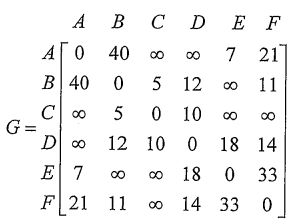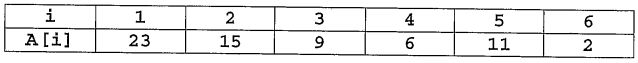lvy>試卷(2022/08/11)

# 102 年 - 102 國立中山大學_碩士班招生考試_電機系(丙組)：資料結構#110205

【非選題】
1.
1.

【題組】 (a) [5 points] Given the size n of the input data, where n is a positive integer, we assume that the running time of a program is O(f(n). State the formal definition of O(f(n)).

【非選題】
2.【題組】

(b) [5 points] Let. Tell us the value ofNote that you will et o points if you just povide the ansver. In other words, you must show your reasons.

【非選題】
3.【題組】(c) [5 points] Given the size n of the input data, where n is a positive integer, we assume that a program requires the running time T1(n) = O( f(n). Derive the function f(n) .

【非選題】
4.【題組】

(d) [5 points] Let n be the radius of a circle C and A(n) be the area of C. Let T2(n)=|sinθ|xlog A(n)= O(f(n) . Derive the function f(n).

【非選題】
5.

2. [5 points] Consider the following function F written in a C-like pseudo-code, which takes an array A of n positive integers and an initially-empty stack S as input parameters:What is the output (returned value) of the function F for the array A= [2, 14, 4, 7, 11, 18, 10, 15, 6, 23, 12, 8]?

【非選題】
6.

3.Consider the following weighted graph G(V,E) presented by the adjacency matrix.【題組】 (1) [10 points] Please find the vertex sequence derived by DFS and BFS respectively. Note that we assume that node A is the root.

【非選題】
7.【題組】(2) [10 points] Please apply Kruskal's algorithm to drive the minimum cost spanning tree. Note that you must show your actions step by step.

【非選題】
8.

4. The array A shown below is used to represent the complete binary tree.Please answer the following three questions:

【題組】 (1) [5 points] Draw the corresponding binary tree T.

【非選題】
9.【題組】(2) [5 points] Is T a max heap? Is T a min heap? Explain your reasons.

【非選題】
10.【題組】(3) [15 points] Perform the following three heap operations sequentially: INSERT (18), INSERT (27) , DELETE on T. Draw the resultant tree after each operation.

【非選題】
11.

5.

【題組】(a) [5 points] Given an unsorted integer array of size n, does the binary search algorithm outperform the sequential search algorithm? Use the big-O notation to justify your answer.

【非選題】
12.【題組】(b) [5 points] Given an integer array A of size n, the following pseudo-code shows the quick-sort algorithm. Note that we assume that all array elements in A are distinct and the function medi um(2) will return the value x in A such that the number of integers that are larger than x is equal to the number of integers that are smaller than x. Besides, we assume that the running time of the function medi um
(A) is O(r). Derive the worst case running time of quicksort
(A) in terms of big-0 notation. (Note that you will get O points if you just give the answer directly.)【非選題】
13.【題組】

(c) [5 points] The following enhanced _quicksort algorithm witten in a C-like pseudo-code tries to first split the array A into three partitions and then recursively sorts the arrays s, M, and L. Note that we carefully design two selection functions, first selection function
(A)and second selection function
(A), to guarantee that min
(A) ＜ x ＜y ＜max
(A), where min
(A) and max
(A) denote the minimum element and the maximum element in array A, respectively. Moreover, we assume that the running time of each selection functions is O(n). Please tell us whether the enhanced quicksort algorithm is more efficient than the quicksort algorithm? Use the big-O notation to justify your answer.【非選題】
14.
6.

【題組】 (1) [5 points] Please convert the infix expression shown below into postfix form. Ax(B/(C-D)+E)xxF-G

【非選題】
15.【題組】

(2) [10 points] Tell us the results of preorder and postorder traversals for the following binary tree.### 懸賞詳解

#### 國二地理上第二次

14、 地理圖五為近年來中國相當普及的商業消費支付方式，此類支付方式的蓬勃發展需要有下列哪一個環境支持？ (A)發達的電子金融系統 (B)民主開明�...